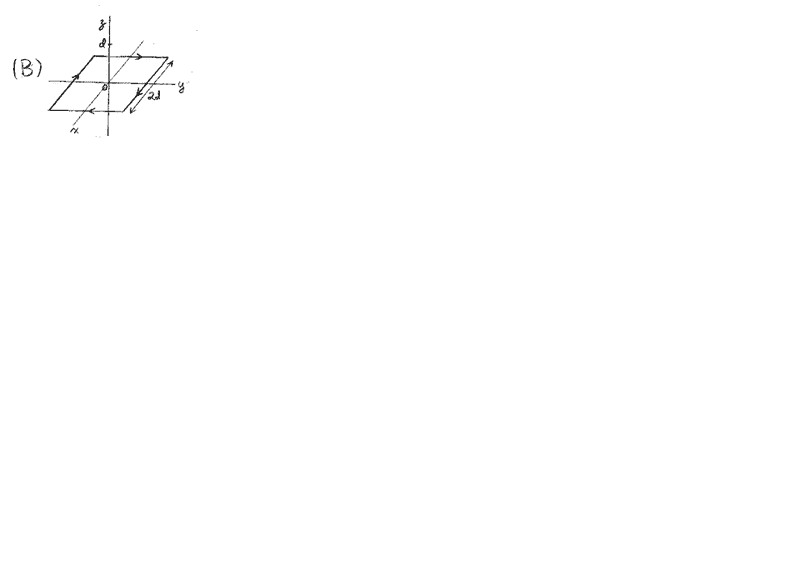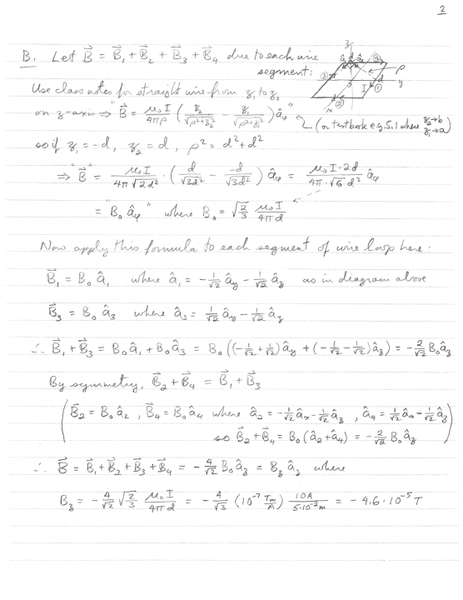# The magnetic field induced by a current loop

Engineering Student
A square loop of wire in the x-y plane, centred on the origin, is made up of four straight segments, each of length 2d=10cm. A current I =10A flows in the wire in the direction shown. Find the magnetic field at z=d on the z-axis.
(See "Diagram" for the diagram of the problem)
Here is the solution provided by the professor:
(See "solution1" for the solution of the problem)
I don't understand how he came up with the expression for unite vectors a1 and a3. Could some one explain this? Thanks.Mentor
A square loop of wire in the x-y plane, centred on the origin, is made up of four straight segments, each of length 2d=10cm. A current I =10A flows in the wire in the direction shown. Find the magnetic field at z=d on the z-axis.
(See "Diagram" for the diagram of the problem)
Here is the solution provided by the professor:
(See "solution1" for the solution of the problem)
I don't understand how he came up with the expression for unite vectors a1 and a3. Could some one explain this? Thanks.
View attachment 99110 View attachment 99111
Welcome to the PF.

All I can see is a small attachment of the figure for the problem. Can you use the UPLOAD button to attach the figure and the solution to a reply? Thanks.Engineering Student
Yes. I uploaded the pictures. You can get the pictures by clicking on the thumbnails.

Homework Helper
Gold Member
Yes. I uploaded the pictures. You can get the pictures by clicking on the thumbnails.
I saw the pictures but feel belabored by your handwriting - I suggest typing in your solution. You can get all sorts of symbols by clicking on "Σ" in the toolbar above.

•berkeman